suppressPackageStartupMessages({
library(ggplot2)
library(ggpattern)
})

## Introduction to the ‘image and ’placeholder’ patterns

• The ‘image’ pattern allows filling a geom with an image from file or URL. It is an “array” pattern that depends on the suggested package magick.

• The ‘placeholder’ pattern fetches images from image placeholder sites. It is an “array” pattern that depends on the suggested package magick and additionally requires an internet connect.

## The image pattern - filling a geom with an image from file or URL

This pattern will load an image from a file or URL (pattern_filename) and display it within the geom.

Because the image will most likely not exactly cover the area of the geom, there are options to specify how the image is used to fill the region.

After expanding to cover the area, the image is then clipped to the boundary of the geom.

The files/URLs are loaded with magick::image_read which means that transparent images and SVGs are supported.

### image options

Aesthetic Description Default
pattern_filename Image filename or URL ’’
pattern_type Image scaling type ‘fit’
pattern_scale Extra scaling 1
pattern_gravity Position of image within area ‘center’
pattern_filter Filter to use when scaling ‘lanczos’
pattern_alpha Alpha NA
pattern_aspect_ratio Override aspect ratio NA
pattern_key_scale_factor Additional scale factor for legend 1
pattern_type Description pattern_scale pattern_gravity
squish distort the image to cover the bounding box of the region
fit scale the image such that either the width or the height of the image fits in the bounding box. Yes
expand scale the image beyond the bounding box and crop it such that the image fully covers the width and the height of the region
none position a single image in the region without attempting to scale to the bounding box size Yes Yes
tile repeat the image to cover the bounding box. Yes

Note: not all combinations of aesthetics are useful e.g. if pattern_type = 'fit' is specified, then pattern_scale has no effect.

### Example Data

These examples use some of the built-in images in the ggpattern package.

#~~~~~~~~~~~~~~~~~~~~~~~~~~~~~~~~~~~~~~~~~~~~~~~~~~~~~~~~~~~~~~~~~~~~~~~~~~~~~
# filenames of images
#~~~~~~~~~~~~~~~~~~~~~~~~~~~~~~~~~~~~~~~~~~~~~~~~~~~~~~~~~~~~~~~~~~~~~~~~~~~~~
logo_filename   <- system.file("img", "Rlogo.png" , package="png")
magpie_filename <- system.file("img", "magpie.jpg", package="ggpattern")
bug_filename    <- system.file("img", "bug.jpg"   , package="ggpattern")

seamless1 <- system.file("img", "seamless1.jpg"   , package="ggpattern")
seamless2 <- system.file("img", "seamless2.jpg"   , package="ggpattern")
seamless3 <- system.file("img", "seamless3.jpg"   , package="ggpattern")

#~~~~~~~~~~~~~~~~~~~~~~~~~~~~~~~~~~~~~~~~~~~~~~~~~~~~~~~~~~~~~~~~~~~~~~~~~~~~~
# Plotting data
#~~~~~~~~~~~~~~~~~~~~~~~~~~~~~~~~~~~~~~~~~~~~~~~~~~~~~~~~~~~~~~~~~~~~~~~~~~~~~
df1 <- data.frame(
trt      = c("a", "b", "c"),
outcome  = c(2.3, 1.9, 3.2),
gravity  = c('South', 'North', 'West'),
filltype = c('squish', 'fit' , 'expand'),
scale    = c(1, 2, 0.5),
filename = c(logo_filename, magpie_filename, bug_filename),
stringsAsFactors = FALSE
)
Example Data
trt outcome gravity filltype scale filename
a 2.3 South squish 1.0 /usr/local/lib/R/site-library/png/img/Rlogo.png
b 1.9 North fit 2.0 /usr/local/lib/R/site-library/ggpattern/img/magpie.jpg
c 3.2 West expand 0.5 /usr/local/lib/R/site-library/ggpattern/img/bug.jpg

### Example: pattern = 'image' - No Attempt at filling the area (pattern_type = 'none')

If pattern_type = 'none' then no attempt is made at snugly filling the area. Instead a single copy of the image is scaled (pattern_scale) and placed (pattern_gravity) in the geom area.

if (require("magick")) {

ggplot(df1, aes(trt, outcome)) +
geom_col_pattern(
aes(
fill            = trt,
pattern_gravity = I(gravity),
pattern_scale   = I(scale)
),
pattern          = 'image',
pattern_filename = logo_filename,
pattern_type     = 'none',
colour           = 'black'
) +
theme_bw(15) +
labs(
title    = "ggpattern::geom_col_pattern()",
subtitle = "pattern = 'image', pattern_type = 'none'"
) +
theme(legend.key.size = unit(1.5, 'cm')) +
coord_fixed(ratio = 1/2)

}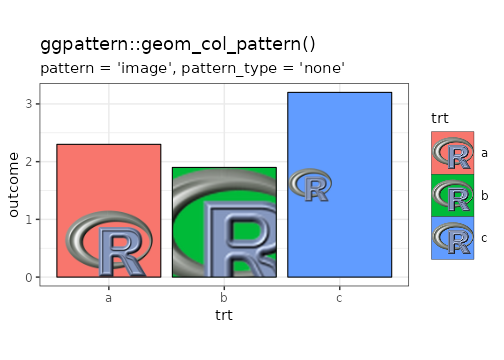plot of chunk none

### Example: pattern = 'image' - Covering the area (pattern_type = 'fit', 'squish', 'expand')

In the plot below, 3 different methods are used to cover the area of the geom. From left-to-right:

• pattern_type = 'squish' to force the image to cover the bounding box of the geom.
• pattern_type = 'fit' to force the image to cover the width (or height) yet still remain fully visible. pattern_gravity = 'North' floats the image to the top.
• pattern_type = 'expand scales the image (while preserving aspect ratio) such that the entire geom is covered.pattern_gravity = ‘West’ anchors the image to the left side of the geom.
if (require("magick")) {

ggplot(df1, aes(trt, outcome)) +
geom_col_pattern(
aes(
fill            = trt,
pattern_gravity = I(gravity),
pattern_type    = I(filltype)
),
pattern          = 'image',
colour           = 'black',
pattern_filename = logo_filename,

) +
theme_bw(15) +
labs(
title    = "ggpattern::geom_col_pattern()",
subtitle = "pattern = 'image'"
) +
# theme(legend.key.size = unit(1.5, 'cm')) +
theme(legend.position = 'none') +
coord_fixed(ratio = 1/2)

}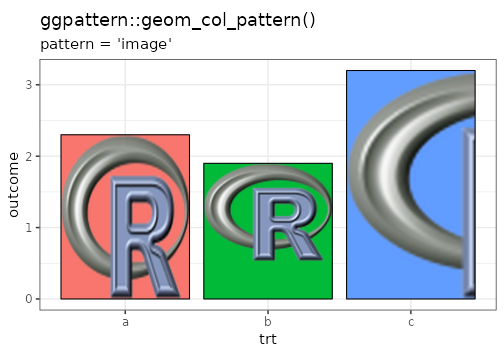plot of chunk covering

### Example: pattern = 'image' - Tiling (pattern_type = 'tile')

When tiled, the image is replicated in order to cover the entire area of the geom. In this example, pattern_scale is also applied to the image so that it appears at different sizes.

if (require("magick")) {

ggplot(df1, aes(trt, outcome)) +
geom_col_pattern(
aes(
fill            = trt,
pattern_gravity = I(gravity),
pattern_scale   = I(scale)
),
pattern          = 'image',
pattern_type     = 'tile',
pattern_filename = logo_filename,
colour           = 'black'
) +
theme_bw(15) +
labs(
title    = "ggpattern::geom_col_pattern()",
subtitle = "pattern = 'image', pattern_type = 'tile'"
) +
# theme(legend.key.size = unit(1.5, 'cm')) +
theme(legend.position = 'none') +
coord_fixed(ratio = 1/2)

}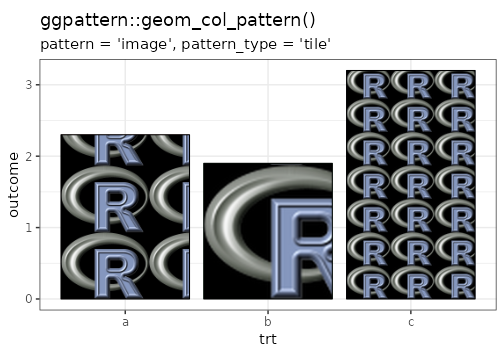plot of chunk tiling

### Example: pattern = 'image' - Tiling with seamless patterns

By choosing a seamlessly tiling image, then a tiled fill will not have visible discontinuities.

if (require("magick")) {

ggplot(mtcars) +
geom_density_pattern(
aes(
x = mpg,
pattern_filename = as.factor(cyl)
),
pattern      = 'image',
pattern_type = 'tile'
) +
theme_bw(15) +
theme(legend.key.size = unit(1.5, 'cm')) +
labs(
title    = "ggpattern::geom_density_pattern()",
subtitle = "pattern = 'image', pattern_type = 'tile'"
) +
scale_pattern_filename_manual(values = c(4 = seamless1, 6 = seamless2, 8 = seamless3)) +
coord_fixed(ratio = 80)

}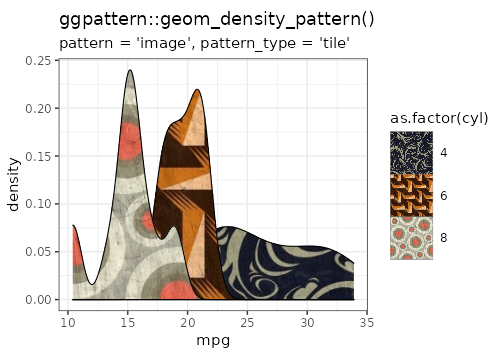plot of chunk tiling-seamless

### Example: pattern = 'image' - Tiling with seamless patterns (with scaling)

if (require("magick")) {

ggplot(mtcars) +
geom_density_pattern(
aes(
x = mpg,
pattern_filename = as.factor(cyl)
),
pattern       = 'image',
pattern_type  = 'tile',
pattern_scale = 0.5
) +
theme_bw(15) +
theme(legend.key.size = unit(2, 'cm')) +
labs(
title    = "ggpattern::geom_density_pattern()",
subtitle = "pattern = 'image', pattern_type = 'tile'"
) +
scale_pattern_filename_manual(values = c(4 = seamless1, 6 = seamless2, 8 = seamless3)) +
coord_fixed(ratio = 80)

}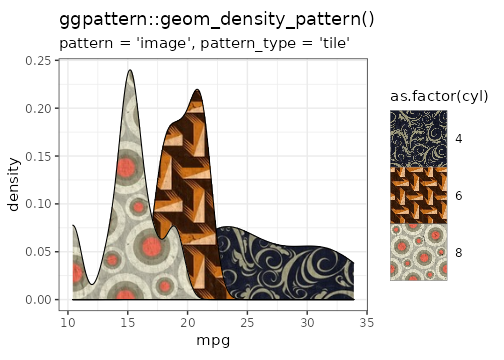plot of chunk tiling-seamless-scaling

### Example: pattern = 'image' - Tiling with fit to width (of element bounding box)

In a common case, the image to be tiled should be first scaled to the width of the bar to be tiled and then tiling appears like a vertical stacking. This is useful for bar charts.

Specify pattern_scale = -1 to fit the width of the geom, and pattern_scale = -2 to fit the height of the geom.

if (require("magick")) {

ggplot(df1, aes(trt, outcome)) +
geom_col_pattern(
aes(
fill             = trt,
pattern_filename = I(filename)
),
pattern          = 'image',
pattern_type     = 'tile',
pattern_scale    = -1,
colour           = 'black'

) +
theme_bw(15) +
labs(
title    = "ggpattern::geom_col_pattern()",
subtitle = "pattern = 'image', pattern_type = 'tile',\npattern_scale = -1"
) +
theme(legend.position = 'none') +
coord_fixed(ratio = 1)

}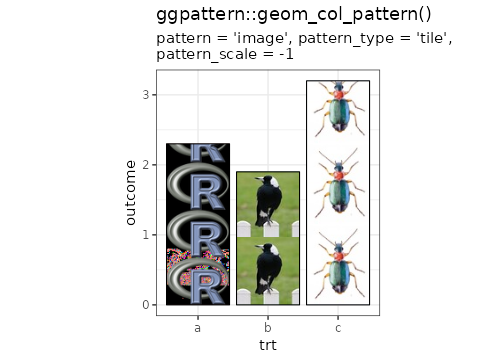plot of chunk tiling-fit

## placeholder - Filling with an image placeholder

Array-based patterns allow the user to specify an RGBA that should be displayed in the geom.

Getting the correct sized image such that it takes up the full space without being distorted can be a time-consuming task.

The placeholder pattern takes out this drudgery by fetching exactly the correct sized image to fit the space. These images come from image placeholder sites which are most often used by web-developers as a stand-in for a final image while they are developing a new site.

### placeholder options

Aesthetic Description Default
pattern_type Image source ‘kitten’
pattern_alpha Alpha NA
pattern_aspect_ratio Override aspect ratio NA
pattern_key_scale_factor Additional scale factor for legend 1

### placeholder option pattern_type

The following is a list of all the pattern_type values which are valid. If unspecified or unknown, then ggpattern will use pattern_type = 'kitten'.

If you would like only greyscale images, append bw to the name, e.g. to display only black and white kittens use pattern_type = 'kittenbw'.

Click the link to see an example of each placeholder generator

All placeholder names are available in gridpattern::names_placeholder.

### Example Data

df1 <- data.frame(
trt     = c("a", "b", "c"),
outcome = c(2.3, 1.9, 3.2),
stringsAsFactors = FALSE
)

### Example: pattern = 'placeholder' - pattern_type = 'bear'

if (require("magick")) {

ggplot(df1, aes(trt, outcome)) +
geom_col_pattern(
aes(fill = trt),
pattern      = 'placeholder',
pattern_type = 'bear',
colour       = 'black'
) +
theme_bw(15) +
labs(
title    = "ggpattern::geom_col_pattern()",
subtitle = "pattern='placeholder', pattern_type='bear'"
) +
theme(legend.position = 'none') +
coord_fixed(ratio = 1/2)

}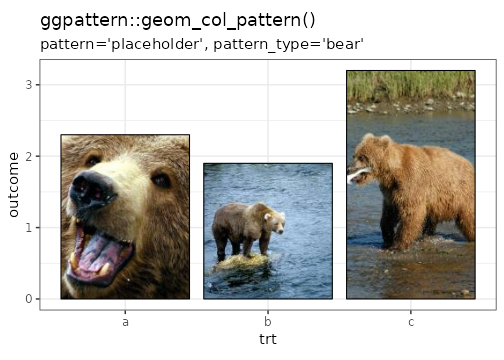plot of chunk bear

### Example: pattern = 'placeholder' - pattern_type = 'picsum'

if (require("magick")) {

ggplot(mtcars) +
geom_density_pattern(
aes(x = mpg, group = as.factor(cyl)),
pattern      = 'placeholder',
pattern_type = 'picsum'
) +
theme_bw(15) +
theme(legend.position = 'none') +
labs(
title    = "ggpattern::geom_col_pattern()",
subtitle = "pattern='placeholder', pattern_type='picsum'"
) +
coord_fixed(ratio = 80)

}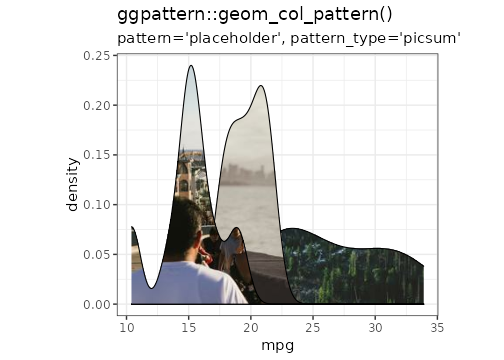plot of chunk picsum

### Example: pattern = 'placeholder' - pattern_type = 'dummy'

if (require("magick")) {

ggplot(df1, aes(trt, outcome)) +
geom_col_pattern(
aes(fill = trt),
pattern       = 'placeholder',
pattern_type  = 'dummy',
colour        = 'black'
) +
theme_bw(15) +
labs(
title    = "ggpattern::geom_col_pattern()",
subtitle = "pattern='placeholder', pattern_type='dummy'"
) +
theme(legend.position = 'none') +
coord_fixed(ratio = 1/2)

}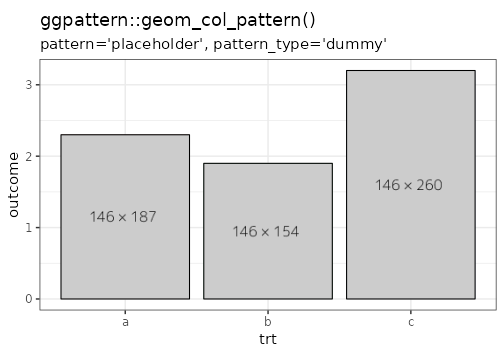plot of chunk dummy

### Example: pattern = 'placeholder' - pattern_type = 'kitten'

if (require("magick")) {

df2 <- data.frame(
group = factor(c("Cool", "But", "Use", "Less"), levels = c("Cool", "But", "Use", "Less")),
value = c(10, 20, 30, 40)
)

ggplot(df2, aes(x="", y = value, pattern_angle = group))+
geom_bar_pattern(
pattern      = 'placeholder',
pattern_type = 'kitten',
pattern_aspect_ratio = 1,
width        = 1,
stat         = "identity",
colour       = 'white',
size         = 2
) +
coord_polar("y", start=0) +
theme_void(15) +
theme(legend.position = 'none') +
labs(
title    = "ggpattern::geom_col_pattern()",
subtitle = "pattern='placeholder', pattern_type='kitten'"
)

}`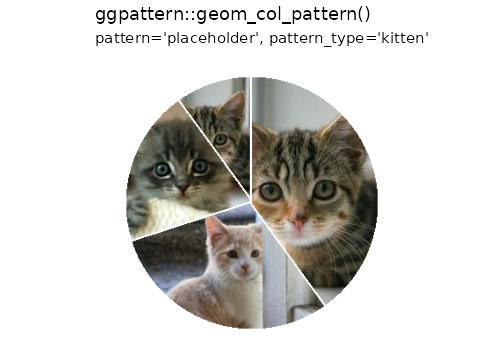plot of chunk kitten